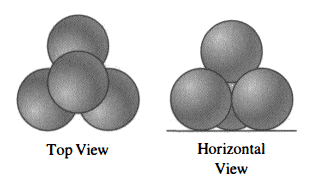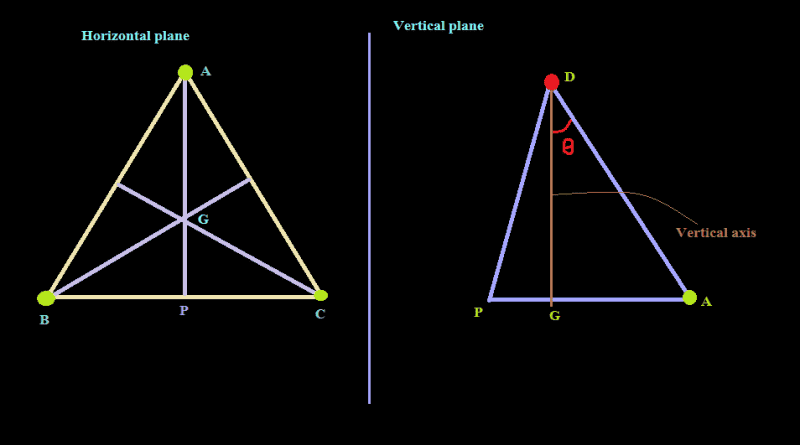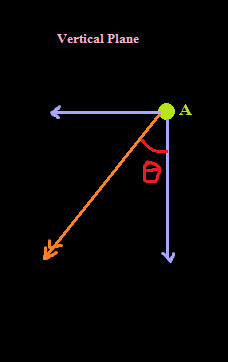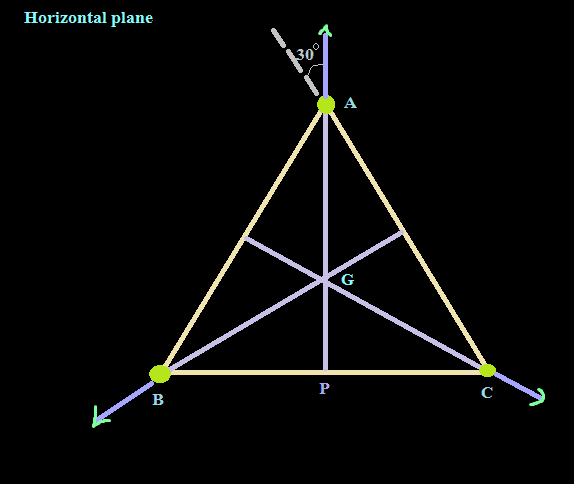# Problem on tension

## Homework Statement[/B]
An ornament for a courtyard at a World's Fair is to be made up of four identical, frictionless metal spheres, each weighing ##2\sqrt{6}## ton-wts.The spheres are to be arranged as shown, with three resting on horizontal surface and touching each other; the fourth is to rest freely on the other three. The bottom three are kept from separating by spot welds at the points of contact with each other. Allowing for a factor of safety of 3, how much tension must the spot welds withstand?

## The Attempt at a Solution

At first, I calculated the angle between the vertical axis and the line segment which connects the centres of the top and bottom spheres. Let, the angle be ##\theta##.;In the picture, A, B , C and D are the centers of the spheres. D is the centre of the top sphere. Let the radius of a sphere be ##R## .
So, AB = BC = CA = AD = 2R ;
Applying pythagorean theorem for APC triangle, ## AP = \sqrt{3} R ##
As, G is the centroid, AG : GP = 2 : 1 ;
So, ## AG = \frac{2R}{\sqrt{3}} ##
From, the triangle AGD, ##sin \theta = \frac{AG}{AD} = \frac{1}{\sqrt{3}} ##
and, ## tan \theta = \frac{1}{\sqrt{2}} ##
The vertically downward (component) force of the top sphere on each of the bottom spheres will be
##= \frac{1}{3} \cdot 2 \sqrt{6} = \frac {2\sqrt{2}}{\sqrt{3}} ##

Now, let's consider the force on the bottom sphere having centre A :So the vertical component is ## \frac {2\sqrt{2}}{\sqrt{3}} ##
And so, horizontal component becomes ## = \frac {2\sqrt{2}}{\sqrt{3}} \cdot tan \theta = \frac{2}{\sqrt{3}}##
Now, look at the forces on the bottom spheres in the horizontal plane.This horizontal force on A, can be divided into two components, along CA and BA ;
To, get the tension in AC, I took the component along AC which is ##= \frac{1}{2} \frac{2}{\sqrt{3}} cos 30 = \frac{1}{2} ##
As, the safety factor is 3, the tension becomes ##\frac{3}{2}##;
But it doesn't match the answer.

TSny
Homework Helper
Gold Member
Your work looks good to me all the way to the last part where you are considering the horizontal forces on the bottom spheres. Draw a free body diagram of one of the bottom spheres showing all of the horizontal force components acting on the sphere.

Last edited:
CWatters
Homework Helper
Gold Member
Check the line that begins...

And so, horizontal component becomes...

When you resolve a force into two orthogonal components the original force vector is the hypotenuse not the side of the triangle.

Chestermiller
Mentor
I confirm your result for the horizontal force. For the horizontal force balance, I get 2Tcos(30)=(horizontal force). This gives T = 2/3. If I multiply by the factor of safety, I get 2.

Chet

I confirm your result for the horizontal force. For the horizontal force balance, I get 2Tcos(30)=(horizontal force). This gives T = 2/3. If I multiply by the factor of safety, I get 2.